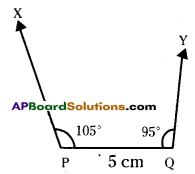# AP Board 7th Class Maths Solutions Chapter 9 Construction of Triangles InText Questions

AP State Syllabus AP Board 7th Class Maths Solutions Chapter 9 Construction of Triangles InText Questions and Answers.

## AP State Syllabus 7th Class Maths Solutions 9th Lesson Construction of Triangles InText QuestionsTry This

Question 1.
Construct a triangle with the same measurements given in above example taking PQ as base. Are the triangles congruent ? (Page No. 183)
Solution:
PQ = 4 cm, QR = 5 cm. RP = 7cm.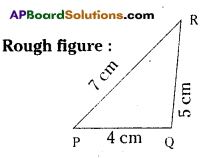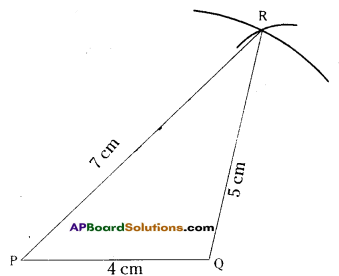Step -1 : Draw a rough sketch of the triangle and label it with the given measurements.
Step – 2 : Draw a line segment PQ of length 4 cm.
Step – 3 : With centre P, drawn an arc of radius 7 cm.
Step – 4 : Since R is at a distance of
5 cm from Q. draw another arc from Q with radius 5 cm such that it intersects first arc at R.
Step – 5 : Join P, R and Q, R. The required APQR is constructed.
The two triangles are congruent by Side – Side – Side (SSS) criterion for congruence.Question 2.
Construct a ΔPET, PE = 4.5 cm, ET = 5.4 cm and TP = 6.5 cm in your notebook. Now construct ΔABC, AB = 5.4 cm, BC = 4.5 cm and CA = 6.5 cm on a piece of paper. Cut it out and place it on the figure you have constructed in your notebook. Are the triangles congruent ? Write your answer using mathematical notation. (Page No. 183)
Solution:
ΔPET, PE = 4.5 cm, ET = 5.4 cm, TP = 6.5 cm.
Step – 1 : Draw a rough sketch of the triangle and label it with the given measurements.
Step – 2 : Draw a line segment PE of length 4.5 cm.
Step – 3 : With centre P, draw an arc of radius 6.5 cm.
Step – 4 : Draw another arc from E with radius – 5. 4 cm such that it intersets first arc at T.
Step – 5 : Join P, T and E, T. The required APET is constructed.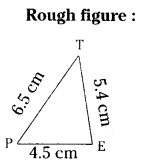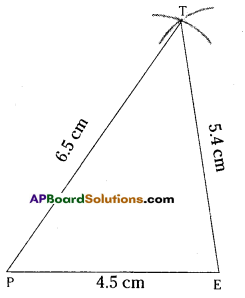ΔABC, AB = 5.4 cm, BC = 4.5 cm and CA = 6.5
Step – 1 : Draw a rough sketch of the
triangle and label it with the given measurements.
Step – 2 : Draw a line segment AB of length 5.4 cm.
Step – 3 : With centre A, draw an arc of radius 6.5 cm.
Step – 4 : Draw another arc from B
with radius 4.5 cm such that it intersects first arc at C.
Step – 5 : Join A, C and B,C. The required AABC is constructed.
If we place the ΔABC on ΔPET, the triangles are congruent. This is because,
AB = TE
AC = PT
BC = PE
∴ ΔABC ≅ ΔTEP (SSS criteria)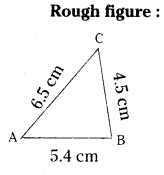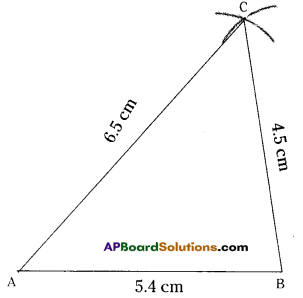Question 3.
Sushanth prepared a problem. Construct ΔXYZ in which XY = 2 cm, YZ = 8 cm and XZ = 4 cm. He also draw the , rough sketch as shown in figure (i).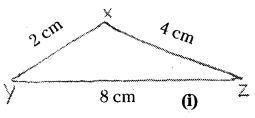Reading the problem, Srija told Sushanth that it would not be possible to draw a triangle with the given measurements. However, Sushanth started to draw the diagram as shown in figure (ii).Check whether Sushanth can draw the triangle. If not, why ?
Discuss with your firends. What property of triangles supports Srija’s idea ? (Page No. 184)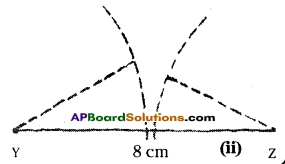Solutuion:
XY = 2 cm, YZ = 8 cm, XZ = 4 cm
Sushanth can not draw the triangle. This is because, in a triangle, the sum of any two sides of a triangle is greater than its third side. But in this case, sum of the two sides is not greater than the third side.
That is XY + XZ ≯ YZ
2 + 4 ≯ YZ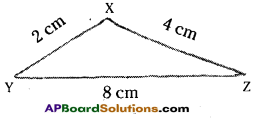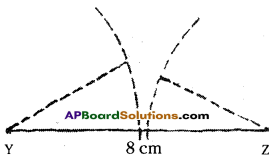So, it is not possible to construct a triangle with the given measurements.Question 4.
Construct a triangle with angles 105° and 95° and a side of length of your choice. Could you construct the triangle ? Discuss and justify.  (Page No. 187)
Solution:
Let PQ = 5 cm, ∠P = 105°, ∠Q = 95°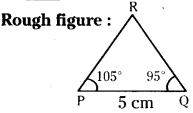We cannot construct a triangle with these measurements. This is because, the sum of three angles in a triangle is 180°. But the sum of the given two angles itself is greater than 180°.
That is 105° + 95° = 200° > 180°
So, it is not possible to construct a triangle with angles 105° and 95°.